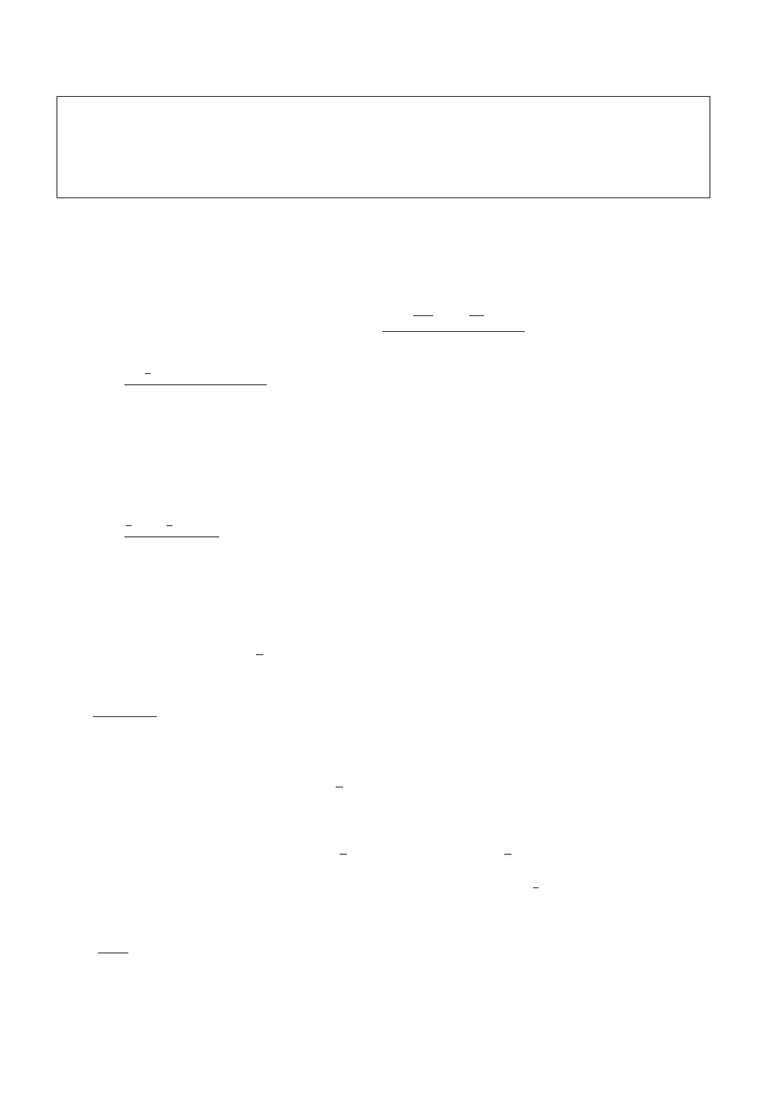# Quiz1A-sol.pdf

754 views3 pagesMAT102F - Introduction to Mathematical Proofs - Fall 2012 - UTM
Quiz 1 (Version A) - September 21, 2012 - Solutions
1. Complete the following sentences: (2 marks)
No explanation is need for this question.
(a) The geometric mean of 4 and 9 is 4·9 = 36 = 6 and their arithmetic mean is
1
2·(4 + 9) = 6.5 .
(b) A glass contains 5 ounces of wine and 3 ounces of water (mixed uniformly). If we remove
2 ounces of liquid from the glass and pour it into an empty cup, then the cup will contain
5
8·2 = 5
4= 1.25 ounces of wine.
2. Given a positive number b, under what conditions does there exist a rectangular carpet with
perimeter 2band area b
Solution:
Denote the dimensions of the carpet by xand y. The given information implies that
xy =b
2and 2x+ 2y= 2b .
The second equation implies that y=bxand then the ﬁrst equation becomes
x(bx) = b
2x2b·x+b
2= 0 .
This quadratic equation has a real solution if and only if (b)24·b
20, or equivalently: b2,
and that is the required condition.
(Note: From the quadratic formula we can conclude that if b2, then the solution(s) will be positive,
and thus no additional conditions are needed.)
2
Unlock document

This preview shows page 1 of the document.
Unlock all 3 pages and 3 million more documents.